## Saturday, 9 August 2014

### CHAPTER 5 - Introduction to Plane

In the chapter on Vectors, we have already learnt how to write the equations for a plane, in different forms. In this section, we will extend that discussion and learn how to write the equation of a plane in three dimensional coordinates form.
The general vector equation of a plane is of the form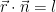$\vec r \cdot \vec n = l$ :$l$ is a constant
where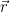$\vec r$ is the variable vector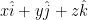$x\hat i + y\hat j + z\hat k$ representing any point on the plane, while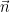$\vec n$ is a fixed vector, say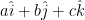$a\hat i + b\hat j + c\hat k$ which is perpendicular to the plane. Thus, the equation of the plane can be written as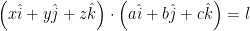$\left( {x\hat i + y\hat j + z\hat k} \right) \cdot \left( {a\hat i + b\hat j + c\hat k} \right) = l$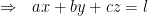$\Rightarrow \,\,\,\, ax + by + cz = l$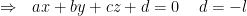$\Rightarrow\,\,\,\,{ax + by + cz + d = 0}\,\,\,\,\,\,\,d = - l$
This is the most general equation of a plane in coordinate form. Note that this equation of the plane contains only three arbitrary constants, for, it can be written as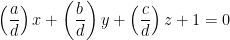$\left( {\dfrac{a}{d}} \right)x + \left( {\dfrac{b}{d}} \right)y + \left( {\dfrac{c}{d}} \right)z + 1 = 0$$\Rightarrow \,\,\,\, {\lambda _1}x + {\lambda _2}y + {\lambda _3}z + 1 = 0$
Thus, three independent constraints are sufficient to uniquely determine a plane. For example, three non collinear points are sufficient to uniquely determine the plane passing through them.
 Example: 9
 Write the equation of an arbitrary plane passing through the point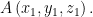$A\left( {{x_1},{y_1},{z_1}} \right).$
 Solution: 9
Let us denote the position vector of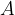$A$ by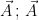$\vec A\,;\,\vec A$ is therefore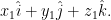${x_1}\hat i + {y_1}\hat j + {z_1}\hat k.$Now, assume that the normal to the plane is$\vec n = a\hat i + b\hat j + c\hat k,$ where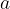$a$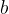$b$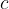$c$are variable :Thus, for any variable point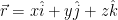$\vec r = x\hat i + y\hat j + z\hat k$ on the plane, since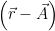$\left( {\vec r - \vec A} \right)$ is perpendicular to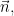$\vec n,$ we have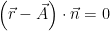$\left( {\vec r - \vec A} \right) \cdot \vec n = 0$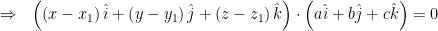$\Rightarrow \,\,\,\, \left( {\left( {x - {x_1}} \right)\hat i + \left( {y - {y_1}} \right)\hat j + \left( {z - {z_1}} \right)\hat k} \right) \cdot \left( {a\hat i + b\hat j + c\hat k} \right) = 0$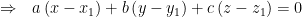$\Rightarrow \,\,\,\, a\left( {x - {x_1}} \right) + b\left( {y - {y_1}} \right) + c\left( {z - {z_1}} \right) = 0$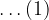$\ldots(1)$
This is the required equation of an arbitrary plane through the point$A({x_1},{y_1},{z_1}).$
We could have arrived at this equation alternatively as follows: we assume the general equation of a plane which is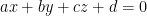$ax + by + cz + d = 0$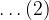$\ldots(2)$
If this passes through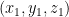$\left( {{x_1},{y_1},{z_1}} \right)$ we have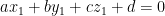$a{x_1} + b{y_1} + c{z_1} + d = 0$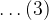$\ldots(3)$
By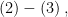$\left( 2 \right) - \left( 3 \right),$ we arrive at the same equation as in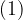$(1)$.